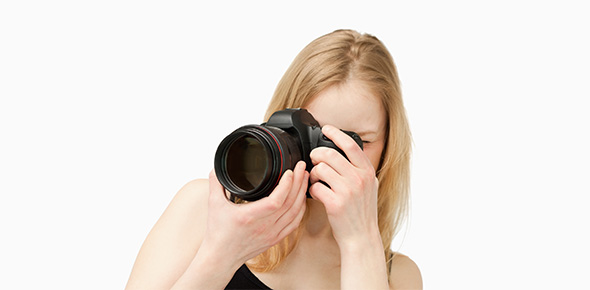# Cinematography Exam II (Timed)

25 Questions | Total Attempts: 66Settings7 MINS

• 1.
500ASA + ND6 + 80A = ___ASA
• 2.
250ASA + ND9 + 85 = ___ASA
• 3.
640ASA + ND3 + 85 = ___ASA
• 4.
3200ASA + ND9 + 80A = ___ASA
• 5.
50 ISO + FLB + 85 = ___ASA
• 6.
4000ASA + ND6 + 80A + ___ASA
• 7.
640ASA + 85 + ND3 = ___ISO
• 8.
100ASA + 85 + ND9 = ___ASA
• 9.
Click those which are TRUE.
• A.

50ASA is a DAYLIGHT film stock

• B.

100ASA is DAYLIGHT film stock

• C.

500ASA is a TUNGSTEN film stock

• D.

200ASA is a DAYLIGHT film stock

• E.

250ASA is a DAYLIGHT film stock

• 10.
What is the colour temp of candle light?
• A.

1,200K

• B.

1,400K

• C.

1,800K

• D.

2,000K

• E.

2,800K

• 11.
What is the colour temp of sunrise/sunset?
• A.

1,200K

• B.

2,400K

• C.

3,200K

• D.

2,000K

• E.

2,200K

• 12.
What is the colour temp of a household bulb?
• A.

2,100K

• B.

2,600K

• C.

2,800K

• D.

2,200K

• E.

2,500K

• 13.
What is the colour temp of tungsten light?
• A.

3,300K

• B.

3,600K

• C.

3,200K

• D.

3,000K

• E.

3,800K

• 14.
What is the colour temp of late afternoon?
• A.

4,300K

• B.

4,600K

• C.

4,200K

• D.

4,500K

• E.

4,800K

• 15.
What is the colour temp of mid day?
• A.

5,600K

• B.

5,300K

• C.

5,400K

• D.

5,500K

• E.

5,900K

• 16.
What is the colour temp of of a overcast day?
• A.

11,000K

• B.

10,000K

• C.

12,000K

• D.

15,000K

• E.

100,000K

• 17.
___mm is a "normal" lens on a 16mm camera
• 18.
___mm is a "normal" lens on a 1/2 inch CCD. (HD)
• 19.
___mm is a "normal" lens on a 2/3 inch CCD. (HD)
• 20.
___mm is a "normal" lens on a 35mm camera
• 21.
Your using a 8:1 lighting ratio.  Key: 2.8,  Fill: ___
• 22.
Your using a 4:1 lighting ratio, Key: __ Fill: 2.8
• 23.
Your using a 2:1 lighting ratio, Key: 4 Fill: __
• 24.
Your using a __:__ lighting ratio, Key: 5.6  Fill: 1.4
• 25.
Your using a 4:1 lighting ratio, Key: 2  Fill: __
Related TopicsBack to top